# Bending

﻿
Bending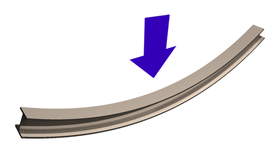Bending of an I-beam

In engineering mechanics, bending (also known as flexure) characterizes the behavior of a slender structural element subjected to an external load applied perpendicularly to a longitudinal axis of the element. The structural element is assumed to be such that at least one of its dimensions is a small fraction, typically 1/10 or less, of the other two. When the length is considerably longer than the width and the thickness, the element is called a beam. A closet rod sagging under the weight of clothes on clothes hangers is an example of a beam experiencing bending. On the other hand, a shell is a structure of any geometric form where the length and the width are of the same order of magnitude but the thickness of the structure (known as the 'wall') is considerably smaller. A large diameter, but thin-walled, short tube supported at its ends and loaded laterally is an example of a shell experiencing bending.

In the absence of a qualifier, the term bending is ambiguous because bending can occur locally in all objects. To make the usage of the term more precise, engineers refer to the bending of rods, the bending of beams, the bending of plates, the bending of shells and so on.

## Quasistatic bending of beams

A beam deforms and stresses develop inside it when a transverse load is applied on it. In the quasistatic case, the amount of bending deflection and the stresses that develop are assumed not to change over time. In a horizontal beam supported at the ends and loaded downwards in the middle, the material at the over-side of the beam is compressed while the material at the underside is stretched. There are two forms of internal stresses caused by lateral loads:

• Shear stress parallel to the lateral loading plus complementary shear stress on planes perpendicular to the load direction;
• Direct compressive stress in the upper region of the beam, and direct tensile stress in the lower region of the beam.

These last two forces form a couple or moment as they are equal in magnitude and opposite in direction. This bending moment resists the sagging deformation characteristic of a beam experiencing bending. The stress distribution in a beam can be predicted quite accurately even when some simplifying assumptions are used.

### Euler-Bernoulli bending theoryElement of a bent beam: the fibers form concentric arcs, the top fibers are compressed and bottom fibers stretched.Bending moments in a beam

In the Euler-Bernoulli theory of slender beams, a major assumption is that 'plane sections remain plane'. In other words, any deformation due to shear across the section is not accounted for (no shear deformation). Also, this linear distribution is only applicable if the maximum stress is less than the yield stress of the material. For stresses that exceed yield, refer to article plastic bending. At yield, the maximum stress experienced in the section (at the furthest points from the neutral axis of the beam) is defined as the flexural strength.

The Euler-Bernoulli equation for the quasistatic bending of slender, isotropic, homogeneous beams of constant cross-section under an applied transverse load q(x) is$EI~\cfrac{\mathrm{d}^4 w(x)}{\mathrm{d} x^4} = q(x)$

where E is the Young's modulus, I is the area moment of inertia of the cross-section, and w(x) is the deflection of the neutral axis of the beam.

After a solution for the displacement of the beam has been obtained, the bending moment (M) and shear force (Q) in the beam can be calculated using the relations$M(x) = -EI~\cfrac{\mathrm{d}^2 w}{\mathrm{d} x^2} ~;~~ Q(x) = \cfrac{\mathrm{d}M}{\mathrm{d}x}$

Simple beam bending is often analyzed with the Euler-Bernoulli beam equation. The conditions for using simple bending theory are :

1. The beam is subject to pure bending. This means that the shear force is zero, and that no torsional or axial loads are present.
2. The material is isotropic and homogeneous.
3. The material obeys Hooke's law (it is linearly elastic and will not deform plastically).
4. The beam is initially straight with a cross section that is constant throughout the beam length.
5. The beam has an axis of symmetry in the plane of bending.
6. The proportions of the beam are such that it would fail by bending rather than by crushing, wrinkling or sideways buckling.
7. Cross-sections of the beam remain plane during bending.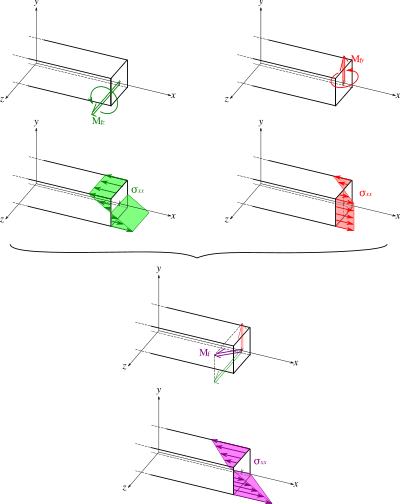Deflection of a beam deflected symmetrically and principle of superposition

Compressive and tensile forces develop in the direction of the beam axis under bending loads. These forces induce stresses on the beam. The maximum compressive stress is found at the uppermost edge of the beam while the maximum tensile stress is located at the lower edge of the beam. Since the stresses between these two opposing maxima vary linearly, there therefore exists a point on the linear path between them where there is no bending stress. The locus of these points is the neutral axis. Because of this area with no stress and the adjacent areas with low stress, using uniform cross section beams in bending is not a particularly efficient means of supporting a load as it does not use the full capacity of the beam until it is on the brink of collapse. Wide-flange beams (I-beams) and truss girders effectively address this inefficiency as they minimize the amount of material in this under-stressed region.

The classic formula for determining the bending stress in a beam under simple bending is:${\sigma}= \frac{M y}{I_x}$

where

• σ is the bending stress
• M - the moment about the neutral axis
• y - the perpendicular distance to the neutral axis
• Ix - the second moment of area about the neutral axis x

### Extensions of Euler-Bernoulli beam bending theory

#### Plastic bending

The equation$\sigma = \tfrac{M y}{I_x}$ is valid only when the stress at the extreme fiber (i.e. the portion of the beam farthest from the neutral axis) is below the yield stress of the material from which it is constructed. At higher loadings the stress distribution becomes non-linear, and ductile materials will eventually enter a plastic hinge state where the magnitude of the stress is equal to the yield stress everywhere in the beam, with a discontinuity at the neutral axis where the stress changes from tensile to compressive. This plastic hinge state is typically used as a limit state in the design of steel structures.

#### Complex or asymmetrical bending

The equation above is only valid if the cross-section is symmetrical. For homogeneous beams with asymmetrical sections, the axial stress in the beam is given by${\sigma_x}(y,z) = -\frac {(M_z~I_y + M_y~I_{yz})} {I_y~I_z - I_{yz}^2}y + \frac {(M_y~I_z + M_z~I_{yz})} {I_y~I_z - I_{yz}^2}z$

where y,z are the coordinates of a point on the cross section at which the stress is to be determined as shown to the right, My and Mz are the bending moments about the y and z centroid axes, Iy and Iz are the second moments of area (distinct from moments of inertia) about the y and z axes, and Iyz is the product of moments of area. Using this equation it is possible to calculate the bending stress at any point on the beam cross section regardless of moment orientation or cross-sectional shape. Note that My,Mz,Iy,Iz,Iyz do not change from one point to another on the cross section.

#### Large bending deformation

For large deformations of the body, the stress in the cross-section is calculated using an extended version of this formula. First the following assumptions must be made:

1. Assumption of flat sections - before and after deformation the considered section of body remains flat (i.e. is not swirled).
2. Shear and normal stresses in this section that are perpendicular to the normal vector of cross section have no influence on normal stresses that are parallel to this section.

Large bending considerations should be implemented when the bending radius ρ is smaller than ten section heights h:

ρ < 10h

With those assumptions the stress in large bending is calculated as:$\sigma = \frac {F} {A} + \frac {M} {\rho A} + {\frac {M} {{I_x}'}}y{\frac {\rho}{\rho +y}}$

where

F is the normal force
A is the section area
M is the bending moment
ρ is the local bending radius (the radius of bending at the current section)
Ix' is the area moment of inertia along the x axis, at the y place (see Steiner's theorem)
y is the position along y axis on the section area in which the stress σ is calculated

When bending radius ρ approaches infinity and y<<ρ, the original formula is back:$\sigma = {F \over A} \pm \frac {My}{I}$.

### Timoshenko bending theoryDeformation of a Timoshenko beam. The normal rotates by an amount θ which is not equal to dw / dx.

In 1921, Timoshenko improved upon the Euler-Bernoulli theory of beams by adding the effect of shear into the beam equation. The kinematic assumptions of the Timoshenko theory are

• normals to the axis of the beam remain straight after deformation
• there is no change in beam thickness after deformation

However, normals to the axis are not required to remain perpendicular to the axis after deformation.

The equation for the quasistatic bending of a linear elastic, isotropic, homogeneous beam of constant cross-section beam under these assumptions is$EI~\cfrac{\mathrm{d}^4 w}{\mathrm{d} x^4} = q(x) - \cfrac{EI}{k A G}~\cfrac{\mathrm{d}^2 q}{\mathrm{d} x^2}$

where I is the area moment of inertia of the cross-section, A is the cross-sectional area, G is the shear modulus, and k is a shear correction factor. For materials with Poisson's ratios (ν) close to 0.3, the shear correction factor for a rectangular cross-section is approximately$k = \cfrac{5 + 5\nu}{6 + 5\nu}$

The rotation ($\varphi(x)$) of the normal is described by the equation$\cfrac{\mathrm{d}\varphi}{\mathrm{d}x} = -\cfrac{\mathrm{d}^2w}{\mathrm{d}x^2} -\cfrac{q(x)}{kAG}$

The bending moment (M) and the shear force (Q) are given by$M(x) = -EI~ \cfrac{\mathrm{d}\varphi}{\mathrm{d}x} ~;~~ Q(x) = kAG\left(\cfrac{\mathrm{d}w}{\mathrm{d}x}-\varphi\right) = -EI~\cfrac{\mathrm{d}^2\varphi}{\mathrm{d}x^2} = \cfrac{\mathrm{d}M}{\mathrm{d}x}$

## Dynamic bending of beams

The dynamic bending of beams, also known as flexural vibrations of beams, was first investigated by Daniel Bernoulli in the late 18th century. Bernoulli's equation of motion of a vibrating beam tended to overestimate the natural frequencies of beams and was improved marginally by Rayleigh in 1877 by the addition of a mid-plane rotation. In 1921 Stephen Timoshenko improved the theory further by incorporating the effect of shear on the dynamic response of bending beams. This allowed the theory to be used for problems involving high frequencies of vibration where the dynamic Euler-Bernoulli theory is inadequate. The Euler-Bernoulli and Timoshenko theories for the dynamic bending of beams continue to be used widely by engineers.

### Euler-Bernoulli theory

The Euler-Bernoulli equation for the dynamic bending of slender, isotropic, homogeneous beams of constant cross-section under an applied transverse load q(x,t) is$EI~\cfrac{\partial^4 w}{\partial x^4} + m~\cfrac{\partial^2 w}{\partial t^2} = q(x,t)$

where E is the Young's modulus, I is the area moment of inertia of the cross-section, w(x,t) is the deflection of the neutral axis of the beam, and m is mass per unit length of the beam.

#### Free vibrations

For the situation where there is no transverse load on the beam, the bending equation takes the form$EI~\cfrac{\partial^4 w}{\partial x^4} + m~\cfrac{\partial^2 w}{\partial t^2} = 0$

Free, harmonic vibrations of the beam can then be expressed as$w(x,t) = \text{Re}[\hat{w}(x)~e^{-i\omega t}] \quad \implies \quad \cfrac{\partial^2 w}{\partial t^2} = -\omega^2~w(x,t)$

and the bending equation can be written as$EI~\cfrac{\mathrm{d}^4 \hat{w}}{\mathrm{d}x^4} - m\omega^2\hat{w} = 0$

The general solution of the above equation is$\hat{w} = A_1\cosh(\beta x) + A_2\sinh(\beta x) + A_3\cos(\beta x) + A_4\sin(\beta x)$

where A1,A2,A3,A4 are constants and$\beta := \left(\cfrac{m}{EI}~\omega^2\right)^{1/4}$

The mode shapes of a cantilevered I-beam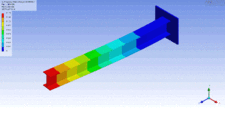1st lateral bending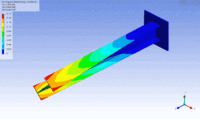1st torsional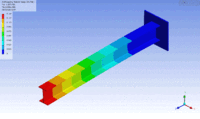1st vertical bending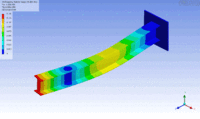2nd lateral bending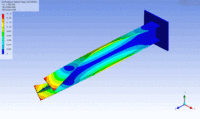2nd torsional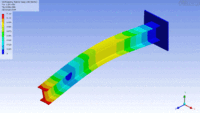2nd vertical bending

### Timoshenko-Rayleigh theory

In 1877, Rayleigh proposed an improvement to the dynamic Euler-Bernoulli beam theory by including the effect of rotational inertia of the cross-section of the beam. Timoshenko improved upon that theory in 1922 by adding the effect of shear into the beam equation. Shear deformations of the normal to the mid-surface of the beam are allowed in the Timoshenko-Rayleigh theory.

The equation for the bending of a linear elastic, isotropic, homogeneous beam of constant cross-section beam under these assumptions is$EI~\cfrac{\partial^4 w}{\partial x^4} + m~\cfrac{\partial^2 w}{\partial t^2} - \left(J + \cfrac{E I m}{k A G}\right)\cfrac{\partial^4 w}{\partial x^2~\partial t^2} + \cfrac{J m}{k A G}~\cfrac{\partial^4 w}{\partial t^4} = q(x,t) + \cfrac{J}{k A G}~\cfrac{\partial^2 q}{\partial t^2} - \cfrac{EI}{k A G}~\cfrac{\partial^2 q}{\partial x^2}$

where$J = \tfrac{mI}{A}$ is the polar moment of inertia of the cross-section, m = ρA is the mass per unit length of the beam, ρ is the density of the beam, A is the cross-sectional area, G is the shear modulus, and k is a shear correction factor. For materials with Poisson's ratios (ν) close to 0.3, the shear correction factor are approximately\begin{align} k &= \tfrac{5 + 5\nu}{6 + 5\nu} \quad \text{rectangular cross-section}\\ &= \tfrac{6 + 12\nu + 6\nu^2}{7 + 12\nu + 4\nu^2} \quad \text{circular cross-section} \end{align}

#### Free vibrations$EI~\cfrac{\mathrm{d}^4 \hat{w}}{\mathrm{d} x^4} + m\omega^2\left(\cfrac{J}{m} + \cfrac{E I}{k A G}\right)\cfrac{\mathrm{d}^2 \hat{w}}{\mathrm{d} x^2} + m\omega^2\left(\cfrac{\omega^2 J}{k A G}-1\right)~\hat{w} = 0$

This equation can be solved by noting that all the derivatives of w must have the same form to cancel out and hence as solution of the form ekx may be expected. This observation leads to the characteristic equation$\alpha~k^4 + \beta~k^2 + \gamma = 0 ~;~~ \alpha := EI ~,~~ \beta := m\omega^2\left(\cfrac{J}{m} + \cfrac{E I}{k A G}\right) ~,~~ \gamma := m\omega^2\left(\cfrac{\omega^2 J}{k A G}-1\right)$

The solutions of this quartic equation are$k_1 = +\sqrt{z_+} ~,~~ k_2 = -\sqrt{z_+} ~,~~ k_3 = +\sqrt{z_-} ~,~~ k_4 = -\sqrt{z_-}$

where$z_+ := \cfrac{-\beta + \sqrt{\beta^2 - 4\alpha\gamma}}{2\alpha} ~,~~ z_-:= \cfrac{-\beta - \sqrt{\beta^2 - 4\alpha\gamma}}{2\alpha}$

The general solution of the Timoshenko-Rayleigh beam equation for free vibrations can then be written as$\hat{w} = A_1~e^{k_1 x} + A_2~e^{-k_1 x} + A_3~e^{k_3 x} + A_4~e^{-k_3 x}$

## Quasistatic bending of platesDeformation of a thin plate highlighting the displacement, the mid-surface (red) and the normal to the mid-surface (blue)

The defining feature of beams is that one of the dimensions is much larger than the other two. A structure is called a plate when it is flat and one of its dimensions is much smaller than the other two. There are several theories that attempt to describe the deformation and stress in a plate under applied loads two of which have been used widely. These are

• the Kirchhoff-Love theory of plates (also called classical plate theory)
• the Mindlin-Reissner plate theory (also called the first-order shear theory of plates)

### Kirchhoff-Love theory of plates

The assumptions of Kirchhoff-Love theory are

• straight lines normal to the mid-surface remain straight after deformation
• straight lines normal to the mid-surface remain normal to the mid-surface after deformation
• the thickness of the plate does not change during a deformation.

These assumptions imply that\begin{align} u_\alpha(\mathbf{x}) & = - x_3~\frac{\partial w^0}{\partial x_\alpha} = - x_3~w^0_{,\alpha} ~;~~\alpha=1,2 \\ u_3(\mathbf{x}) & = w^0(x_1, x_2) \end{align}

where$\mathbf{u}$ is the displacement of a point in the plate and w0 is the displacement of the mid-surface.

The strain-displacement relations are\begin{align} \varepsilon_{\alpha\beta} & = - x_3~w^0_{,\alpha\beta} \\ \varepsilon_{\alpha 3} & = 0 \\ \varepsilon_{33} & = 0 \end{align}

The equilibrium equations are$M_{\alpha\beta,\alpha\beta} + q(x) = 0 ~;~~ M_{\alpha\beta} := \int_{-h}^h x_3~\sigma_{\alpha\beta}~dx_3$

where q(x) is an applied load normal to the surface of the plate.

In terms of displacements, the equilibrium equations for an isotropic, linear elastic plate in the absence of external load can be written as$w^0_{,1111} + 2~w^0_{,1212} + w^0_{,2222} = 0$

In direct tensor notation,$\nabla^2\nabla^2 w = 0$

### Mindlin-Reissner theory of plates

The special assumption of this theory is that normals to the mid-surface remain straight and inextensible but not necessarily normal to the mid-surface after deformation. The displacements of the plate are given by\begin{align} u_\alpha(\mathbf{x}) & = - x_3~\varphi_\alpha ~;~~\alpha=1,2 \\ u_3(\mathbf{x}) & = w^0(x_1, x_2) \end{align}

where φα are the rotations of the normal.

The strain-displacement relations that result from these assumptions are\begin{align} \varepsilon_{\alpha\beta} & = - x_3~\varphi_{\alpha,\beta} \\ \varepsilon_{\alpha 3} & = \cfrac{1}{2}~\kappa\left(w^0_{,\alpha}- \varphi_\alpha\right) \\ \varepsilon_{33} & = 0 \end{align}

where κ is a shear correction factor.

The equilibrium equations are\begin{align} & M_{\alpha\beta,\beta}-Q_\alpha = 0 \\ & Q_{\alpha,\alpha}+q = 0 \end{align}

where$Q_\alpha := \kappa~\int_{-h}^h \sigma_{\alpha 3}~dx_3$

## Dynamic bending of plates

### Dynamics of thin Kirchhoff plates

The dynamic theory of plates determines the propagation of waves in the plates, and the study of standing waves and vibration modes. The equations that govern the dynamic bending of Kirchhoff plates are$M_{\alpha\beta,\alpha\beta} - q(x,t) = J_1~\ddot{w}^0 - J_3~\ddot{w}^0_{,\alpha\alpha}$

where, for a plate with density ρ = ρ(x),$J_1 := \int_{-h}^h \rho~dx_3 ~;~~ J_3 := \int_{-h}^h x_3^2~\rho~dx_3$

and$\ddot{w}^0 = \frac{\partial^2 w^0}{\partial t^2} ~;~~ \ddot{w}^0_{,\alpha\beta} = \frac{\partial^2 \ddot{w}^0}{\partial x_\alpha \partial x_\beta}$

The figures below show some vibrational modes of a circular plate.

Wikimedia Foundation. 2010.

### Look at other dictionaries:

• bending — bénding s. n. Trimis de siveco, 10.08.2004. Sursa: Dicţionar ortografic ﻿ BÉNDING s.n. (muz.) Procedeul alternării arcuşului cu ciupirea coardelor (la contrabas). [< engl. bending]. Trimis de LauraGellner, 13.09.2007. Sursa: DN ﻿ BÉNDING s. n …   Dicționar Român

• bending — bending; un·bending; …   English syllables

• Bending — Bend ing, n. The marking of the clothes with stripes or horizontal bands. [Obs.] Chaucer. [1913 Webster] …   The Collaborative International Dictionary of English

• Bending — Bending. См. Изгиб. (Источник: «Металлы и сплавы. Справочник.» Под редакцией Ю.П. Солнцева; НПО Профессионал , НПО Мир и семья ; Санкт Петербург, 2003 г.) …   Словарь металлургических терминов

• bending — index flexible, malleable, sequacious Burton s Legal Thesaurus. William C. Burton. 2006 …   Law dictionary

• bending — bènding m <N mn nzi> DEFINICIJA glazb. savijanje tona, poseban ukras koji se dobije tehnikom disanja kod nekih instrumenata (usne harmonike) ETIMOLOGIJA engl …   Hrvatski jezični portal

• Bending — Animation. Das oben dargestellte Bending als Tabulatur. In manchen Fällen wird auch Release Bending bei der Rückkehr zum Ursprungston angegeben …   Deutsch Wikipedia

• Bending — Bend Bend, v. t. [imp. & p. p. {Bended} or {Bent}; p. pr. & vb. n. {Bending}.] [AS. bendan to bend, fr. bend a band, bond, fr. bindan to bind. See {Bind}, v. t., and cf. 3d & 4th {Bend}.] 1. To strain or move out of a straight line; to crook by… …   The Collaborative International Dictionary of English

• Bending — This interesting and unusual name is of Medieval English origin and is a dialectal variant of a locational name Benenden from a place so called in Kent. The derivation is from an Old English personal name Bionna with denn , swine pasture, thus… …   Surnames reference

• bending — adj. Bending is used with these nouns: ↑motion …   Collocations dictionary